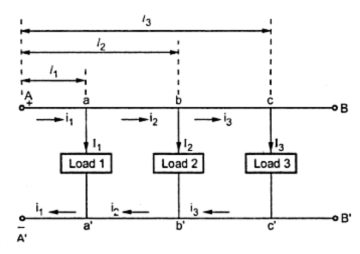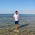### D.C. Distributor With Concentrated Loads

This distributor is further classified as,
1. Fed at one end.
2. Fed at both the ends.
1.1 Concentrated Loads Fed at One End
The Fig. 1 shows a distributor with concentrated loads fed at one end A - A'. The loads are connected at the points a - a', b - b' and c - c'.
Let   1,     and    are the lengths of the sections A - a, A - b and A - c respectively. The load currents are I1, I2 and I3. The currents in various sections of the distributor are i1, i and i3.
From the Fig. 1, applying KCL at various points we can write,Fig. 1

The wire A' B' s the return wire of the distributor.
Let   r' = Resistance per unit length of conductor in Ω
The various voltage drops can be tabulate as,

Applying KVL to any loop point voltages can be calculated.
Now r' is the resistance of single conductor per unit length. And it can be seen that the drops in forward and return conductors are required to be calculated separately and are equal. In practice, the resistance of go and return conductors per unit length is assumed to be r = 2r' and hence two wire distributor can be represented as single wire for calculation purpose, as shown in the Fig. 2.
Now                 r = 2 r'1
r = 2r'
r  = 2r'Fig. 2 Single wire representation

And hence all the drop calculations and load point voltages remain same as before.
The total drop in the distributor is
= r i    r i  (     ) + r i  (     2)
Many times instead of specifying resistances per unit length, actual resistance are specified. The resistance values are of both go and return conductors.
The current loading and voltage drop diagrams are shown in the Fig. 3.Fig. 3  Current loading and voltage drop diagrams

It can be seen that the minimum potential will occur at point c which is farthest from the point A where the distributor is fed at.
1.2 Concentrated Loads Fed at Both End
This type s further classified depending upon the voltage levels at the two ends.
1. Ends fed with equal voltages 2. Ends fed with unequal voltages.
1.2.1 Ends at Equal Voltages
The Fig. 4 shows this type of distributor. The ends A and B are maintained at equal voltages.Fig. 4

Let r1r2r and r are the go and return resistances of the sections Aa, ab, bc and cB respectively.
Let point 'b' be the point of minimum potential.
As we move from point A towards B the potential goes on decreasing and at point b becomes minimum. All the currents between section Ab are supplied by point A. After b the voltage goes on increasing till it becomes feeding voltage at B. All the currents between Bans b are supplied by the point B.
Now the current at minimum potential point b is supplied by both. Let x be supplied by point A while y be supplied by point B. It is obvious that y =  I - x.
As both the points A and B are maintained at same voltage, drop in section Aa must be equal to drop in section Bb.
...               i r +  i2  r  =  i3  r +  i r
...               (I1 + x) r1 + x r   = (I - x) r + (I+ I - x) r
Thus knowing all the currents, x can be calculated and all the voltage drops can be obtained.Fig. 5 Current loading and voltage drop diagrams

The load point where the currents are coming from both the sides of the distributor is the point of minimum potential.
1.2.2 Ends at Unequal Voltages
The Fig. 6 shows this type of distributor. The end s A and B are maintained at different voltages.Fig. 6

Let the resistances of the sections Aa, ab, and cB are r1,  r2,  r and r respectively. Let point 'b' is the point of minimum potential.
In this case also the point b is fed by both the points A and B. The current from point A is x while B it is I2 - x. Now we can write the equation as,
Voltage drop between A and B = Voltage drop over AB.
If voltage of A is is V1 and is greater than voltage of B which is V2 then,
V1 - V2  = drops in all the sections of AB
The same equation can be written as,
V1 - drops over Ab = V2 - drops over Bb
Vi r -  i2  r = V2 - i3  r - i r
V - (I+ x) r1 - xr  = V2 - (x  - x) r - (I+ I-x) r
Solving this equation, as v1 and v2 are known, x can be obtained.Fig. 7 Current loading and voltage drop diagrams

1.Love to read from start to end … you have good information I really like that also your work is appreciative thank you for sharing it with us J

www.schnap.com.au

2.when distributor fed at both ends then there is any shrot circuiting occurs..???

3.